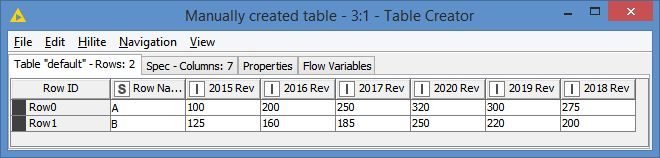# Multiple math formulas?

Is there a node in which I can do multiple math formulas? For example: I have several columns of data, each column in a diff year. I want to calculate the growth rate for each year

``````    2015 Rev           2016  Rev            2017 Rev
``````

A 100 200 250
B 125 160 185

New Columns would be:
2016 Growth: Formula = (2016 Rev - 2015 Rev)/(2016 Rev
2017 Growth: Formula = (2017 Rev - 2016 Rev)/(2017 Rev

Is it possible with a single node? Or do I need multiple Math Formula Nodes?

Check out the column expressions node

2 Likes

Hi @ahortonmilsig and welcome to the Knime Community.

It looks like you have 2 questions:

1. Is there a node in which I can do multiple math formulas
Answer: There are a few ways to do this. If your formulas are going to be nested, for example round(sin(colMin(x, y, z))), this can be done directly in the Math Formula node. However, this can be limited if you need more operations or if you need to temporarily assign some results to some variables, in which case you can use the Column Expressions.

2. Is it possible with a single node (that is applying Math formulas to different columns)? Or do I need multiple Math Formula Nodes?
Answer: You can use the Math Formula (Multi Column) – KNIME Hub to apply the same formula(s) to multiple columns in that 1 node. However you would still be limited to what the Math Formula node can do, except that here, you can apply the formula to all the columns that you choose to, in 1 node.
If you have more complex operations to do, then you have to use the Column Expressions, in which case you would have to loop over each column and apply the Column Expressions for each column inside the loop.
Also, with the Column Expressions, you can define different formulas for different columns as you can define multiple expressions within the same node

Hi @ahortonmilsig , I originally just read your questions without looking at what you were trying to do.

To handle what you are trying to do, you can do this via the Column Expressions as follows:

As you can see, we can define 2 expressions here for the sample data that you gave. 2 Growth column for 3 given years. Each of the 2 expressions will create a new column, so it will add 2 new columns:Now, the limitation with this method is that:
(1) You have to hardcode the column names
(2) You have to create an expression for each of the years for which you want to calculate the Growth

For example, if I have this input data instead:I will get the Growth data only for 2016 and 2017 if I don’t add additional expressions:

I have put together something a bit more dynamic. Nothing needs to be changed whether you have 2 years (you need a minimum of 2 years for this) or however many that you want. In addition, it also properly handle which ever order the year columns are (see in my above input data).

Here’s what the workflow looks like:

Results using the original input data:Results using the input data with more years:

As you can see, the workflow was able to handle the additional years automatically.

Here’s the workflow: Dynamic column pairs calculation.knwf (34.7 KB)

2 Likes